# Fourier on Meshes¶

$\newcommand{\dotp}{\langle #1, #2 \rangle}$ $\newcommand{\enscond}{\lbrace #1, #2 \rbrace}$ $\newcommand{\pd}{ \frac{ \partial #1}{\partial #2} }$ $\newcommand{\umin}{\underset{#1}{\min}\;}$ $\newcommand{\umax}{\underset{#1}{\max}\;}$ $\newcommand{\umin}{\underset{#1}{\min}\;}$ $\newcommand{\uargmin}{\underset{#1}{argmin}\;}$ $\newcommand{\norm}{\|#1\|}$ $\newcommand{\abs}{\left|#1\right|}$ $\newcommand{\choice}{ \left\{ \begin{array}{l} #1 \end{array} \right. }$ $\newcommand{\pa}{\left(#1\right)}$ $\newcommand{\diag}{{diag}\left( #1 \right)}$ $\newcommand{\qandq}{\quad\text{and}\quad}$ $\newcommand{\qwhereq}{\quad\text{where}\quad}$ $\newcommand{\qifq}{ \quad \text{if} \quad }$ $\newcommand{\qarrq}{ \quad \Longrightarrow \quad }$ $\newcommand{\ZZ}{\mathbb{Z}}$ $\newcommand{\CC}{\mathbb{C}}$ $\newcommand{\RR}{\mathbb{R}}$ $\newcommand{\EE}{\mathbb{E}}$ $\newcommand{\Zz}{\mathcal{Z}}$ $\newcommand{\Ww}{\mathcal{W}}$ $\newcommand{\Vv}{\mathcal{V}}$ $\newcommand{\Nn}{\mathcal{N}}$ $\newcommand{\NN}{\mathcal{N}}$ $\newcommand{\Hh}{\mathcal{H}}$ $\newcommand{\Bb}{\mathcal{B}}$ $\newcommand{\Ee}{\mathcal{E}}$ $\newcommand{\Cc}{\mathcal{C}}$ $\newcommand{\Gg}{\mathcal{G}}$ $\newcommand{\Ss}{\mathcal{S}}$ $\newcommand{\Pp}{\mathcal{P}}$ $\newcommand{\Ff}{\mathcal{F}}$ $\newcommand{\Xx}{\mathcal{X}}$ $\newcommand{\Mm}{\mathcal{M}}$ $\newcommand{\Ii}{\mathcal{I}}$ $\newcommand{\Dd}{\mathcal{D}}$ $\newcommand{\Ll}{\mathcal{L}}$ $\newcommand{\Tt}{\mathcal{T}}$ $\newcommand{\si}{\sigma}$ $\newcommand{\al}{\alpha}$ $\newcommand{\la}{\lambda}$ $\newcommand{\ga}{\gamma}$ $\newcommand{\Ga}{\Gamma}$ $\newcommand{\La}{\Lambda}$ $\newcommand{\si}{\sigma}$ $\newcommand{\Si}{\Sigma}$ $\newcommand{\be}{\beta}$ $\newcommand{\de}{\delta}$ $\newcommand{\De}{\Delta}$ $\newcommand{\phi}{\varphi}$ $\newcommand{\th}{\theta}$ $\newcommand{\om}{\omega}$ $\newcommand{\Om}{\Omega}$

This tour explores the use of the eigenvectors of the Laplacian, for filtering and for compression.

In :
addpath('toolbox_signal')


## Fourier Basis on Meshes¶

The Fourier basis are defined as the eigenvector of the Laplacian.

In :
name = 'elephant-50kv';
options.name = name;
n = size(vertex,2);


The combinatorial laplacian is a linear operator (thus a NxN matrix where N is the number of vertices). It depends only on the connectivity of the mesh, thus on face only.

Compute edge list.

In :
E = [faces([1 2],:) faces([2 3],:) faces([3 1],:)];
p = size(E,2);


In :
W = sparse( E(1,:), E(2,:), ones(p,1) );
W = max(W,W');


Compute the combinatorial Laplacian, stored as a sparse matrix.

In :
D = spdiags(sum(W)', 0, n,n);
L = D-W;


The eigenvector of this matrix forms an orthogonal basis of the vector space of signal of NxN values (one real value per vertex). Those functions are the extension of the Fourier oscillating functions to surfaces. For a small mesh (less than 1000) vertices, one can compute this set of vectors using the |eig| functions. For large meshes, one can compute only a small (e.g. 50) number of low pass eigenvectors using the sparse eigenvector extraction procedure, |eigs|.

Compute the eigenvectors.

In :
nb = 80;
opts.disp = 0;
[U,S] = eigs(L,nb,'SM',opts);
S = diag(S);


Order the eigenvector by increasing frequencies.

In :
[S,I] = sort(S, 'ascend');
U = real( U(:,I) );


Plot the eigenvalues. This corresponds to the spectrum of the triangulation. It depends only on the topology of the mesh.

In :
clf;
plot(S); axis('tight');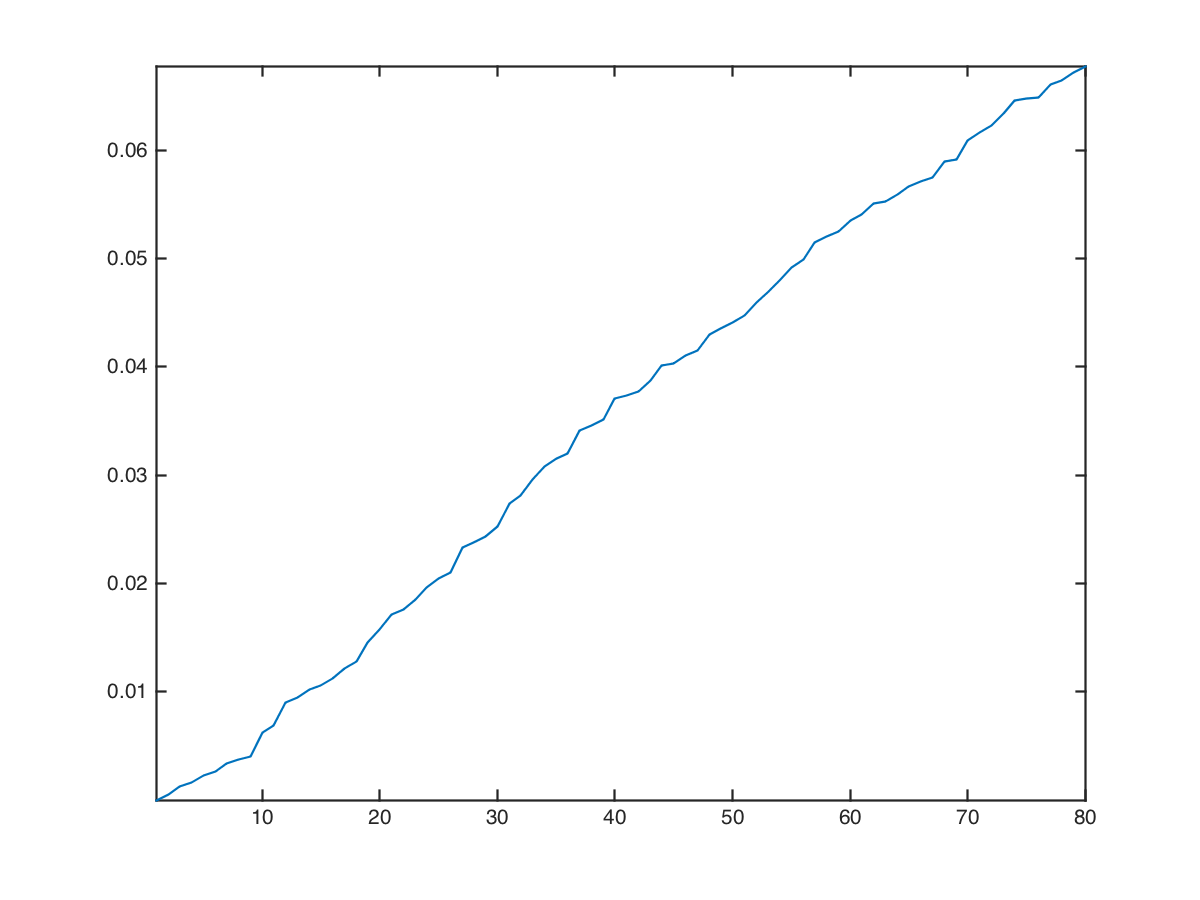Display a sub-set of eigenvectors.

In :
ilist = round(linspace(3,nb, 6));
tau=2.2; % saturation for display
clf;
for i=1:length(ilist)
v = real(U(:,ilist(i)));
v = clamp( v/std(v),-tau,tau );
options.face_vertex_color = v;
subplot(2,3,i);
plot_mesh(vertex,faces,options);
colormap jet(256);
end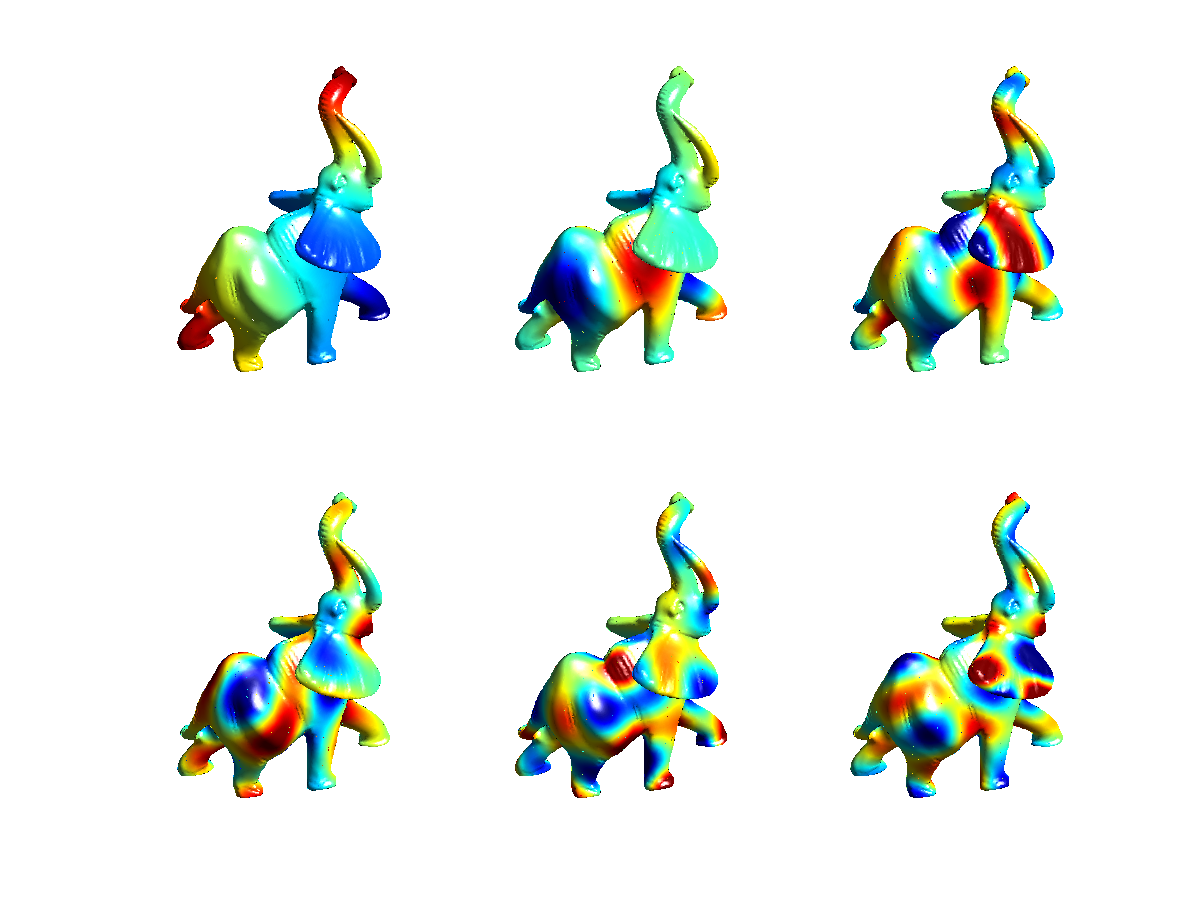## Linear Approximation over the Fourier Domain¶

Linear approximation is obtained by keeping only the low frequency coefficient. This corresponds to a low pass filtering, since high frequency coefficients are removed.

Compute the projection of each coordinate |vertex(i,:)| on the small set of |nb| frequencies.

In :
pvertex = vertex*U;


Display the spectrum pf.

In :
clf;
plot(pvertex'); axis('tight');
legend('X', 'Y', 'Z');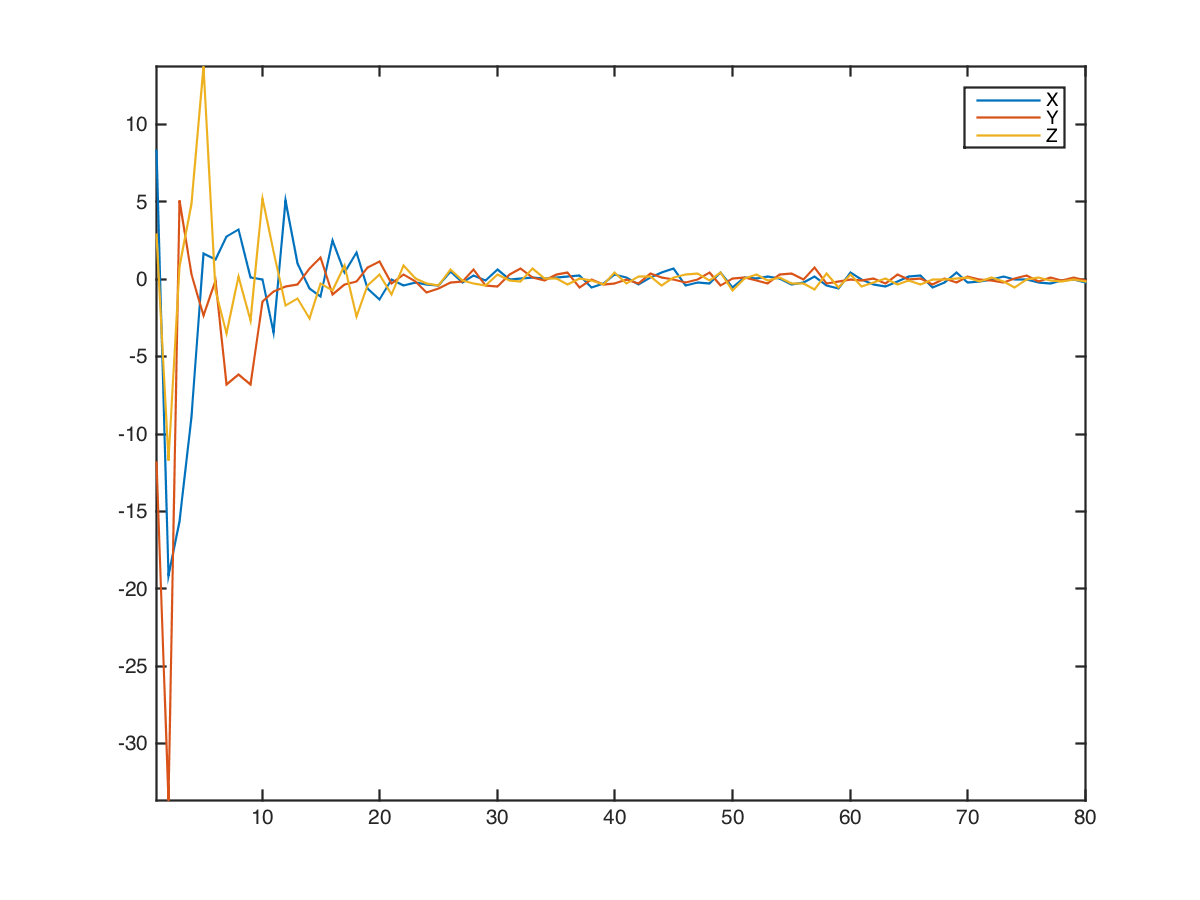Reconstruct the mesh.

In :
vertex1 = pvertex*U';


Compare before and after approximation.

In :
clf;
subplot(1,2,1);
plot_mesh(vertex,faces);
subplot(1,2,2);
plot_mesh(vertex1,faces);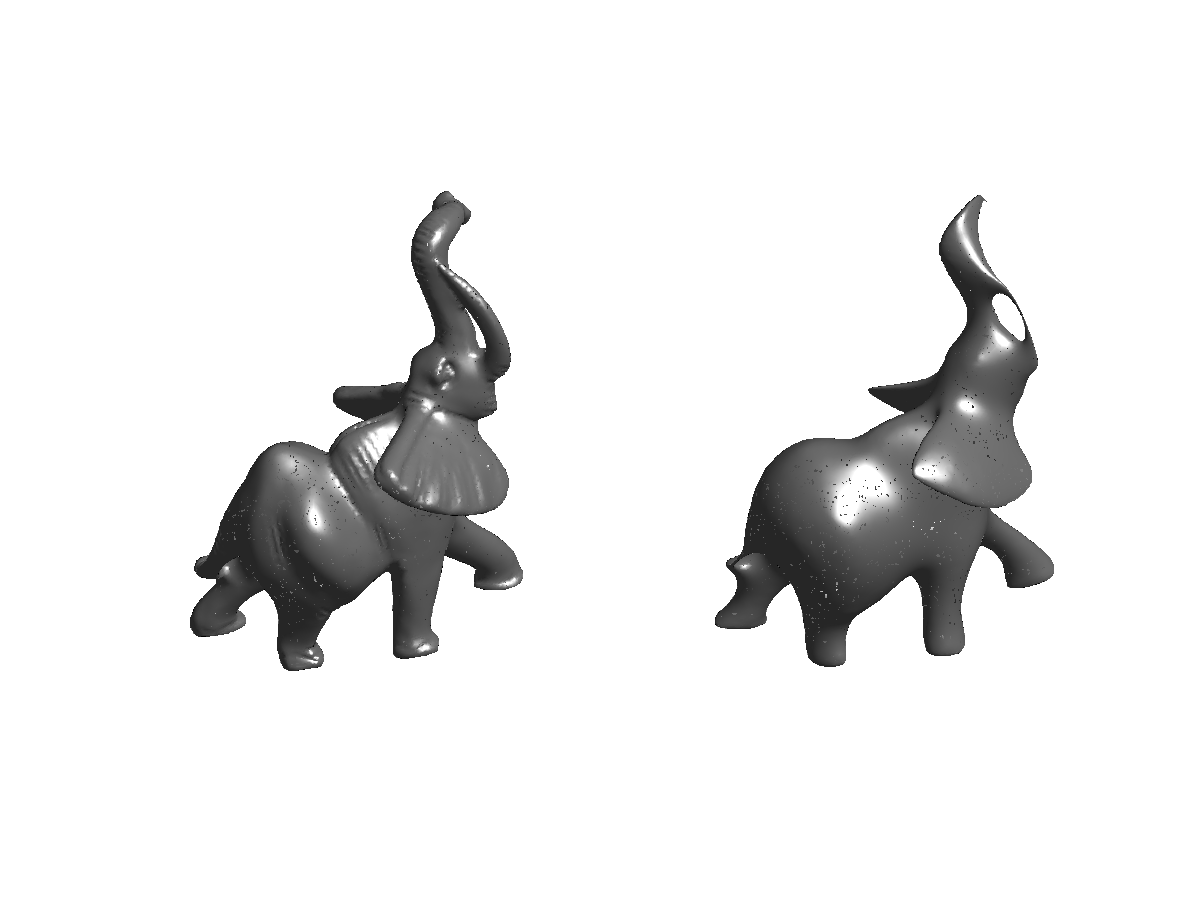Exercise 1

Show the smoothed mesh for an increasing number of Fourier frequencies |nb|.

In :
exo1()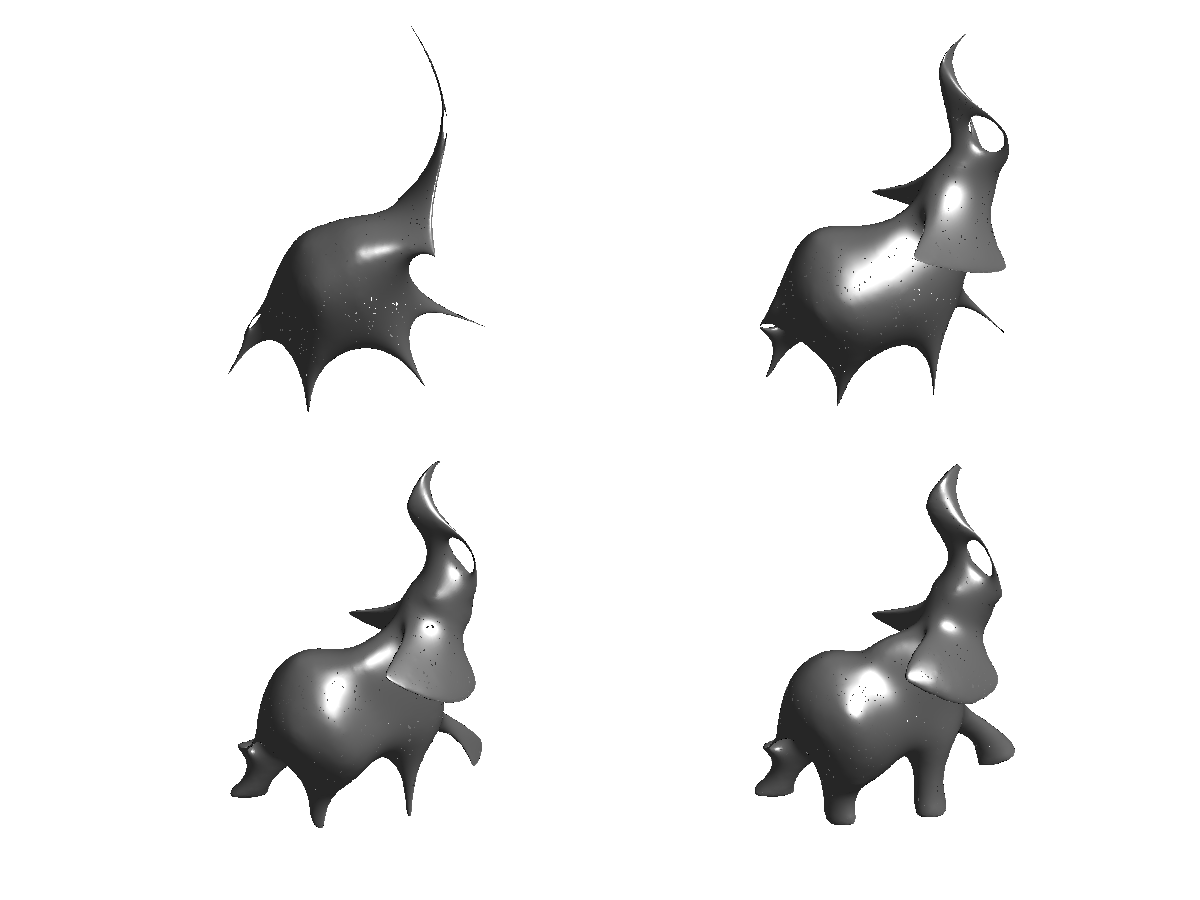In :
%% Insert your code here.


## Non-linear Approximation over the Fourier Domain¶

Non-linear approximation is obtained by keeping the largest magnitude coefficients. It is more efficient than linear approximation since the L2 error is reduced.

We study here the approximation on a small mesh, to be able to compute all the wavelet coefficients.

In :
name = 'venus';
options.name = name;
n = size(vertex,2);


Compute the combinatorial laplacian operator |L| of the mesh.

In :
E = [faces([1 2],:) faces([2 3],:) faces([3 1],:)];
W = sparse( E(1,:), E(2,:), ones(size(E,2),1) );
W = max(W,W');
L = spdiags(sum(W)', 0, n,n) - W;


Compute the full set of eigenvector.

In :
[U,S] = eig(full(L));
S = diag(S);
[S,I] = sort(S, 'ascend');
U = real( U(:,I) );


Plot the eigenvalues.

In :
clf;
plot(S); axis('tight');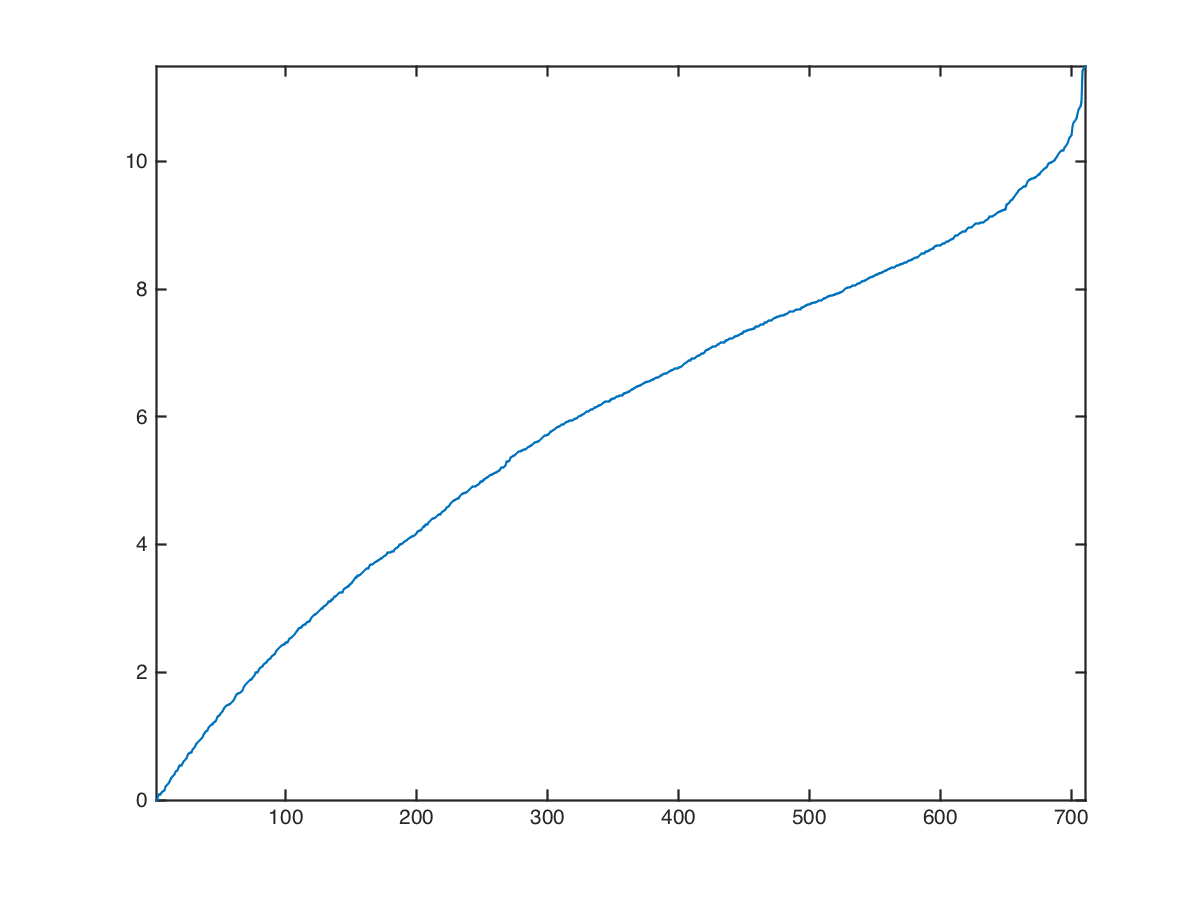Exercise 2

Compute a best |m|-term non-linear approximation whith |m=.1*n|, by hard thresholding the Fourier coefficients using the correct threshold. Compare with linear |m| term approximation (use |m/3| coefficient for each coordinate X/Y/Z). on linear inear isplay

In :
exo2()

Linear:     SNR=22dB
Non-linear: SNR=24.2dB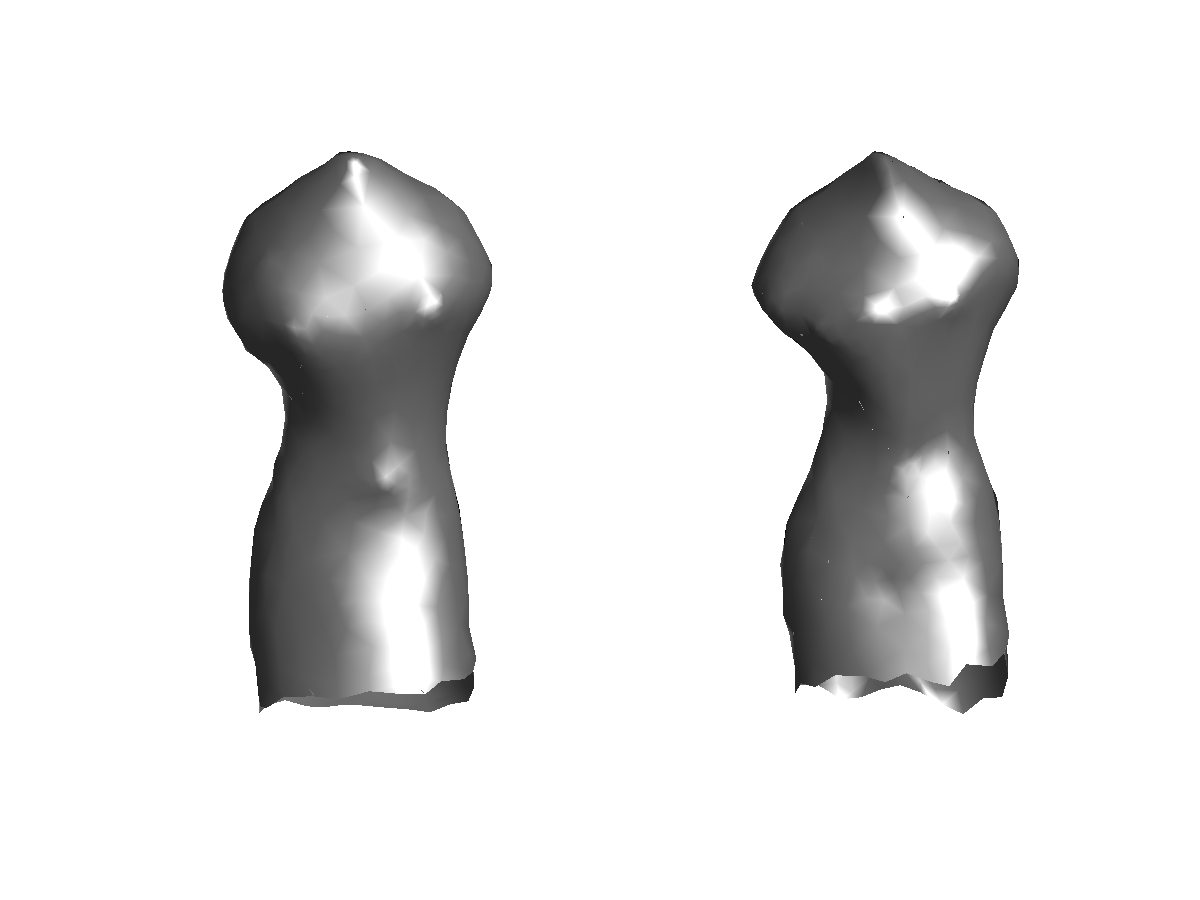In :
%% Insert your code here.


Exercise 3

Compare the rate-distortion curve (log of error as a function of the log of the number of coefficients) for linear and non-linear approximation. onlinear inear ormalize isplay

In :
exo3()